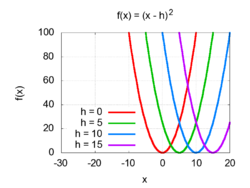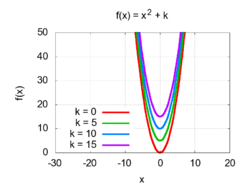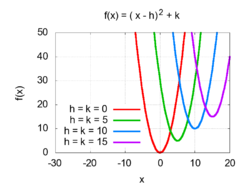# Completing the square

﻿
Completing the square

In elementary algebra, completing the square is a technique for converting a quadratic polynomial of the form$ax^2 + bx + c\,\!$

to the form$a(\cdots\cdots)^2 + \mbox{constant}.\,$

In this context, "constant" means not depending on x. The expression inside the parenthesis is of the form (x − constant). Thus one converts ax2 + bx + c to$a(x - h)^2 + k\,$

and one must find h and k.

Completing the square is used in

In mathematics, completing the square is considered a basic algebraic operation, and is often applied without remark in any computation involving quadratic polynomials.

## Overview

### Background

There is a simple formula in elementary algebra for computing the square of a binomial:$(x + p)^2 \,=\, x^2 + 2px + p^2.\,\!$

For example:\begin{alignat}{2} (x+3)^2 \,&=\, x^2 + 6x + 9 && (p=3)\\[3pt] (x-5)^2 \,&=\, x^2 - 10x + 25\qquad && (p=-5). \end{alignat}

In any perfect square, the number p is always half the coefficient of x, and the constant term is equal to p2.

### Basic example$x^2 + 10x + 28.\,\!$

This quadratic is not a perfect square, since 28 is not the square of 5:$(x+5)^2 \,=\, x^2 + 10x + 25.\,\!$

However, it is possible to write the original quadratic as the sum of this square and a constant:$x^2 + 10x + 28 \,=\, (x+5)^2 + 3.$

This is called completing the square.

### General description$x^2 + bx + c,\,\!$

it is possible to form a square that has the same first two terms:$\left(x+\tfrac{1}{2} b\right)^2 \,=\, x^2 + bx + \tfrac{1}{4}b^2.$

This square differs from the original quadratic only in the value of the constant term. Therefore, we can write$x^2 + bx + c \,=\, \left(x + \tfrac{1}{2}b\right)^2 + k,$

where k is a constant. This operation is known as completing the square. For example:\begin{alignat}{1} x^2 + 6x + 11 \,&=\, (x+3)^2 + 2 \\[3pt] x^2 + 14x + 30 \,&=\, (x+7)^2 - 19 \\[3pt] x^2 - 2x + 7 \,&=\, (x-1)^2 + 6. \end{alignat}

### Non-monic case

Given a quadratic polynomial of the form$ax^2 + bx + c\,\!$

it is possible to factor out the coefficient a, and then complete the square for the resulting monic polynomial.

Example:\begin{align} 3x^2 + 12x + 27 &= 3(x^2+4x+9)\\ &{}= 3\left((x+2)^2 + 5\right)\\ &{}= 3(x+2)^2 + 15 \end{align}

This allows us to write any quadratic polynomial in the form$a(x-h)^2 + k.\,\!$

### Formula

The result of completing the square may be written as a formula. For the general case:$ax^2 + bx + c \;=\; a(x-h)^2 + k,\quad\text{where}\quad h = -\frac{b}{2a} \quad\text{and}\quad k = c - \frac{b^2}{4a}.$

Specifically, when a=1:$x^2 + bx + c \;=\; (x-h)^2 + k,\quad\text{where}\quad h = -\frac{b}{2} \quad\text{and}\quad k = c - \frac{b^2}{4}.$

## Relation to the graphGraphs of quadratic functions shifted to the right by h = 0, 5, 10, and 15.Graphs of quadratic functions shifted upward by k = 0, 5, 10, and 15.Graphs of quadratic functions shifted upward and to the right by 0, 5, 10, and 15.

In analytic geometry, the graph of any quadratic function is a parabola in the xy-plane. Given a quadratic polynomial of the form$(x-h)^2 + k \quad\text{or}\quad a(x-h)^2 + k$

the numbers h and k may be interpreted as the Cartesian coordinates of the vertex of the parabola. That is, h is the x-coordinate of the axis of symmetry, and k is the minimum value (or maximum value, if a < 0) of the quadratic function.

In other words, the graph of the function ƒ(x) = x2 is a parabola whose vertex is at the origin (0, 0). Therefore, the graph of the function ƒ(x − h) = (x − h)2 is a parabola shifted to the right by h whose vertex is at (h, 0), as shown in the top figure. In contrast, the graph of the function ƒ(x) + kx2 + k is a parabola shifted upward by k whose vertex is at (0, k), as shown in the center figure. Combining both horizontal and vertical shifts yields ƒ(x − h) + k = (x − h)2 + k is a parabola shifted to the right by h and upward by k whose vertex is at (hk), as shown in the bottom figure.

Completing the square may be used to solve any quadratic equation. For example:$x^2 + 6x + 5 = 0,\,\!$

The first step is to complete the square:$(x+3)^2 - 4 = 0.\,\!$

Next we solve for the squared term:$(x+3)^2 = 4.\,\!$

Then either$x+3 = -2 \quad\text{or}\quad x+3 = 2,$

and therefore$x = -5 \quad\text{or}\quad x = -1.$

This can be applied to any quadratic equation. When the x2 has a coefficient other than 1, the first step is to divide out the equation by this coefficient: for an example see the non-monic case below.

### Irrational and complex roots

Unlike methods involving factoring the equation, which is only reliable if the roots are rational, completing the square will find the roots of a quadratic equation even when those roots are irrational or complex. For example, consider the equation$x^2 - 10x + 18 = 0.\,\!$

Completing the square gives$(x-5)^2 - 7 = 0,\,\!$

so$(x-5)^2 = 7.\,\!$

Then either$x-5 = -\sqrt{7} \quad\text{or}\quad x-5 = \sqrt{7},\,$

so$x = 5 - \sqrt{7}\quad\text{or}\quad x = 5 + \sqrt{7}. \,$

In terser language:$x = 5 \pm \sqrt{7}.\,$

Equations with complex roots can be handled in the same way. For example:$\begin{array}{c} x^2 + 4x + 5 \,=\, 0 \\[6pt] (x+2)^2 + 1 \,=\, 0 \\[6pt] (x+2)^2 \,=\, -1 \\[6pt] x+2 \,=\, \pm i \\[6pt] x \,=\, -2 \pm i. \end{array}$

### Non-monic case

For an equation involving a non-monic quadratic, the first step to solving them is to divide through by the coefficient of x2. For example:$\begin{array}{c} 2x^2 + 7x + 6 \,=\, 0 \\[6pt] x^2 + \tfrac{7}{2}x + 3 \,=\, 0 \\[6pt] \left(x+\tfrac{7}{4}\right)^2 - \tfrac{1}{16} \,=\, 0 \\[6pt] \left(x+\tfrac{7}{4}\right)^2 \,=\, \tfrac{1}{16} \\[6pt] x+\tfrac{7}{4} = \tfrac{1}{4} \quad\text{or}\quad x+\tfrac{7}{4} = -\tfrac{1}{4} \\[6pt] x = -\tfrac{3}{2} \quad\text{or}\quad x = -2. \end{array}$

## Other applications

### Integration

Completing the square may be used to evaluate any integral of the form$\int\frac{dx}{ax^2+bx+c}$

using the basic integrals$\int\frac{dx}{x^2 - a^2} = \frac{1}{2a}\ln\left|\frac{x-a}{x+a}\right| +C \quad\text{and}\quad \int\frac{dx}{x^2 + a^2} = \frac{1}{a}\arctan\left(\frac{x}{a}\right) +C.$

For example, consider the integral$\int\frac{dx}{x^2 + 6x + 13}.$

Completing the square in the denominator gives:$\int\frac{dx}{(x+3)^2 + 4} \,=\, \int\frac{dx}{(x+3)^2 + 2^2}.$

This can now be evaluated by using the substitution u = x + 3, which yields$\int\frac{dx}{(x+3)^2 + 4} \,=\, \frac{1}{2}\arctan\left(\frac{x+3}{2}\right)+C.$

### Complex numbers

Consider the expression$|z|^2 - b^*z - bz^* + c,\,$

where z and b are complex numbers, z* and b* are the complex conjugates of z and b, respectively, and c is a real number. Using the identity |u|2 = uu* we can rewrite this as$|z-b|^2 - |b|^2 + c , \,\!$

which is clearly a real quantity. This is because\begin{align} |z-b|^2 &{}= (z-b)(z-b)^*\\ &{}= (z-b)(z^*-b^*)\\ &{}= zz^* - zb^* - bz^* + bb^*\\ &{}= |z|^2 - zb^* - bz^* + |b|^2 . \end{align}

As another example, the expression$ax^2 + by^2 + c , \,\!$

where a, b, c, x, and y are real numbers, with a > 0 and b > 0, may be expressed in terms of the square of the absolute value of a complex number. Define$z = \sqrt{a}\,x + i \sqrt{b} \,y .$

Then\begin{align} |z|^2 &{}= z z^*\\ &{}= (\sqrt{a}\,x + i \sqrt{b}\,y)(\sqrt{a}\,x - i \sqrt{b}\,y) \\ &{}= ax^2 - i\sqrt{ab}\,xy + i\sqrt{ba}\,yx - i^2by^2 \\ &{}= ax^2 + by^2 , \end{align}

so$ax^2 + by^2 + c = |z|^2 + c . \,\!$

## Geometric perspectiveConsider completing the square for the equation$x^2 + bx = a.\,$

Since x2 represents the area of a square with side of length x, and bx represents the area of a rectangle with sides b and x, the process of completing the square can be viewed as visual manipulation of rectangles.

Simple attempts to combine the x2 and the bx rectangles into a larger square result in a missing corner. The term (b/2)2 added to each side of the above equation is precisely the area of the missing corner, whence derives the terminology "completing the square". 

## A variation on the technique

As conventionally taught, completing the square consists of adding the third term, v 2 to$u^2 + 2uv\,$

to get a square. There are also cases in which one can add the middle term, either 2uv or −2uv, to$u^2 + v^2\,$

to get a square.

### Example: the sum of a positive number and its reciprocal

By writing\begin{align} x + {1 \over x} &{} = \left(x - 2 + {1 \over x}\right) + 2\\ &{}= \left(\sqrt{x} - {1 \over \sqrt{x}}\right)^2 + 2 \end{align}

we show that the sum of a positive number x and its reciprocal is always greater than or equal to 2. The square of a real expression is always greater than or equal to zero, which gives the stated bound; and here we achieve 2 just when x is 1, causing the square to vanish.

### Example: factoring a simple quartic polynomial

Consider the problem of factoring the polynomial$x^4 + 324 . \,\!$

This is$(x^2)^2 + (18)^2, \,\!$

so the middle term is 2(x2)(18) = 36x2. Thus we get\begin{align} x^4 + 324 &{}= (x^4 + 36x^2 + 324 ) - 36x^2 \\ &{}= (x^2 + 18)^2 - (6x)^2 =\text{a difference of two squares} \\ &{}= (x^2 + 18 + 6x)(x^2 + 18 - 6x) \\ &{}= (x^2 + 6x + 18)(x^2 - 6x + 18) \end{align}

(the last line being added merely to follow the convention of decreasing degrees of terms).

Wikimedia Foundation. 2010.

### Look at other dictionaries:

• completing the square — Math. a method, usually of solving quadratic equations, by which a quadratic expression, as x2 4x + 3, is written as the sum or difference of a perfect square and a constant, x2 4x + 4 + 3 4 = (x 2)2 1, by addition and subtraction of appropriate… …   Universalium

• completing the square — Math. a method, usually of solving quadratic equations, by which a quadratic expression, as x2 4x + 3, is written as the sum or difference of a perfect square and a constant, x2 4x + 4 + 3 4 = (x 2)2 1, by addition and subtraction of appropriate… …   Useful english dictionary

• The World Ends with You — Left to right, Joshua, Neku, Beat (above), Shiki, and Rhyme Developer(s) Square Enix Jupiter …   Wikipedia

• Square Kilometre Array — The Square Kilometre Array (SKA) is a radio telescope in development which will have a total collecting area of approximately one square kilometre. [ [http://www.skatelescope.org/ Square Kilometre Array] website.] It will operate over a wide… …   Wikipedia

• The Sims 3 — Developer(s) The Sims Studio Publisher(s) Electronic Arts …   Wikipedia

• The Legend of Zelda (video game) — The Legend of Zelda North American box art Developer(s) Nintendo EAD Publisher(s) Ninten …   Wikipedia

• The Irish (in Countries Other Than Ireland) —     The Irish (in countries other than Ireland)     † Catholic Encyclopedia ► The Irish (in countries other than Ireland)     I. IN THE UNITED STATES     Who were the first Irish to land on the American continent and the time of their arrival are …   Catholic encyclopedia

• The Elder Scrolls IV: Oblivion — The Elder Scrolls IV: Oblivion …   Wikipedia

• The New York Times crossword puzzle — is a daily puzzle found in The New York Times and online at the paper s website. It is also syndicated to more than 300 other newspapers and journals. The puzzle is created by various freelance constructors and is edited by Will Shortz. The… …   Wikipedia

• The Amazing Race 10 — Season run September 17, 2006 – December 10, 2006 Filming dates May 27, 2006 – June 24, 2006 No. of episodes 13 Winning team Tyler Denk James Branaman Continents visited …   Wikipedia